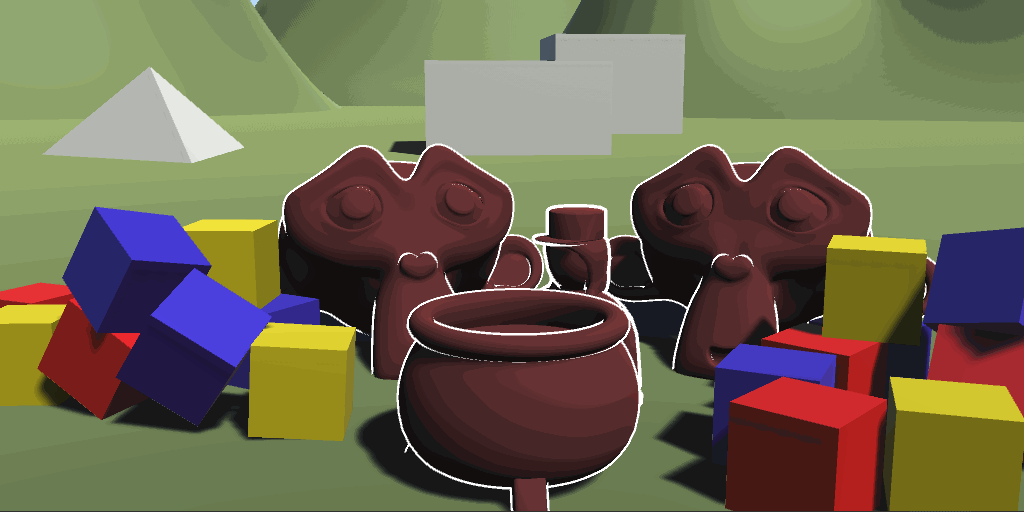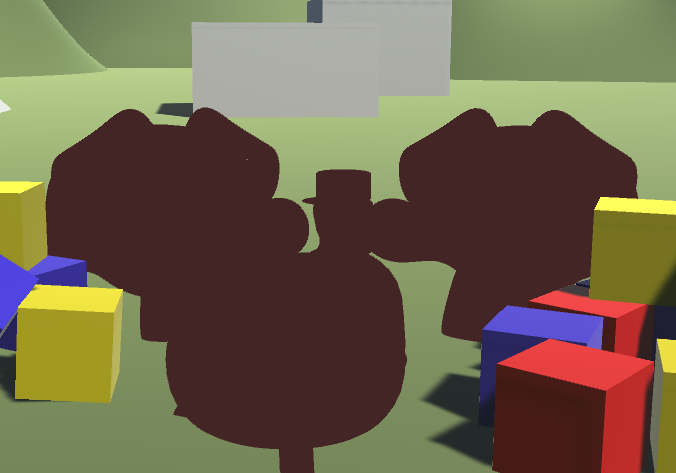## Summary

So far we only ever wrote a color to the screen once per shader (or let unity generate multiple passes for us via surface shaders). But we have the possibility to draw our mesh multiple times in a single shader. A great way to use this is to draw outlines. First we draw our object as usual and then we draw it again, but we change the vertices a bit so it’s only visible around the original object, drawing a outline.

To understand this Tutorial it’s best if you understood surface shaders.

The first version of this shader will be based on the simple textured unlit shader.## Outlines for Unlit Shaders

We already have a shader pass in this shader, so we just duplicate that for now. Because we’re writing the same information twice, this doesn’t change how the shader looks though.

``````//The second pass where we render the outlines
Pass{
CGPROGRAM

//include useful shader functions
#include "UnityCG.cginc"

//define vertex and fragment shader
#pragma vertex vert
#pragma fragment frag

//texture and transforms of the texture
sampler2D _MainTex;
float4 _MainTex_ST;

//tint of the texture
fixed4 _Color;

//the object data that's put into the vertex shader
struct appdata{
float4 vertex : POSITION;
float2 uv : TEXCOORD0;
};

//the data that's used to generate fragments and can be read by the fragment shader
struct v2f{
float4 position : SV_POSITION;
float2 uv : TEXCOORD0;
};

v2f vert(appdata v){
v2f o;
//convert the vertex positions from object space to clip space so they can be rendered
o.position = UnityObjectToClipPos(v.vertex);
o.uv = TRANSFORM_TEX(v.uv, _MainTex);
return o;
}

fixed4 frag(v2f i) : SV_TARGET{
fixed4 col = tex2D(_MainTex, i.uv);
col *= _Color;
return col;
}

ENDCG
}
``````

The next change is to set up our properties and variables. This second pass will only write a simple color to the screen so we don’t need the texture. we just need the outline color and the outline thickness. We put the properties in the properties area at the top like usual. It’s important that we put the new variables in the second pass though.

``````//show values to edit in inspector
Properties{
_OutlineColor ("Outline Color", Color) = (0, 0, 0, 1)
_OutlineThickness ("Outline Thickness", Range(0,.1)) = 0.03

_Color ("Tint", Color) = (0, 0, 0, 1)
_MainTex ("Texture", 2D) = "white" {}
}
``````
``````//color of the outline
fixed4 _OutlineColor;
//thickness of the outline
float _OutlineThickness;
``````

The next step is to rewrite our fragment shader to use the new variable instead of a texture. We can simply return the color without any additional calculations in there.

``````//the fragment shader
fixed4 frag(v2f i) : SV_TARGET{
return _OutlineColor;
}
``````

Because we don’t read from a texture in this pass, we can also ignore the uv coordinates, so we remove them from our input struct, our vertex to fragment struct and we stop passing them between the structs in the vertex shader.

``````//the object data that's available to the vertex shader
struct appdata{
float4 vertex : POSITION;
};

//the data that's used to generate fragments and can be read by the fragment shader
struct v2f{
float4 position : SV_POSITION;
};

v2f vert(appdata v){
v2f o;
//convert the vertex positions from object space to clip space so they can be rendered
o.position = UnityObjectToClipPos(position);
return o;
}
``````With those changes, we can see in the editor that the objects now simply have the color the outlines should have. That’s because our second pass simply draws over everything the first pass has drawn. That’s a thing we’re going to fix later though.

Before that we ensure that the outlines are actually outside of the base object. For that we simply expand them along the their normals. That means we need the normals in our input struct, then we simply add them to the position of the vertices. We also normalize the normals and multiply them with the outline thickness to make the outlines as thick as we want them to be.

``````//the object data that's available to the vertex shader
struct appdata{
float4 vertex : POSITION;
float3 normal : NORMAL;
};
``````
``````//the vertex shader
v2f vert(appdata v){
v2f o;
//calculate the position of the expanded object
float3 normal = normalize(v.normal);
float3 outlineOffset = normal * _OutlineThickness;
float3 position = v.vertex + outlineOffset;
//convert the vertex positions from object space to clip space so they can be rendered
o.position = UnityObjectToClipPos(position);

return o;
}
``````With this we can now adjust the thickness of our hull, but it’s still hiding the base objects. The fix for that is that we don’t draw the front of the hull. Usually when we render objects we only draw the front because of performance reasons (you might have looked inside a object before and were able to look outside, that’s why). For this we can now invert that and only draw the backside. That means we can still see the object because we can look into the hull and we can see the hull behinde the object because it’s bigger than the object itself.

To tell unity to not render the frontsides of objects we add the Cull Front attribute to the hull pass outside of the hlsl area.

``````//The second pass where we render the outlines
Pass{
Cull Front
``````And with this we have the outlines how we want them.

### Source

``````Shader "Tutorial/19_InvertedHull/Unlit"{
//show values to edit in inspector
Properties{
_OutlineColor ("Outline Color", Color) = (0, 0, 0, 1)
_OutlineThickness ("Outline Thickness", Range(0,.1)) = 0.03

_Color ("Tint", Color) = (0, 0, 0, 1)
_MainTex ("Texture", 2D) = "white" {}
}

//the material is completely non-transparent and is rendered at the same time as the other opaque geometry
Tags{ "RenderType"="Opaque" "Queue"="Geometry"}

//The first pass where we render the Object itself
Pass{
CGPROGRAM

//include useful shader functions
#include "UnityCG.cginc"

//define vertex and fragment shader
#pragma vertex vert
#pragma fragment frag

//texture and transforms of the texture
sampler2D _MainTex;
float4 _MainTex_ST;

//tint of the texture
fixed4 _Color;

//the object data that's put into the vertex shader
struct appdata{
float4 vertex : POSITION;
float2 uv : TEXCOORD0;
};

//the data that's used to generate fragments and can be read by the fragment shader
struct v2f{
float4 position : SV_POSITION;
float2 uv : TEXCOORD0;
};

v2f vert(appdata v){
v2f o;
//convert the vertex positions from object space to clip space so they can be rendered
o.position = UnityObjectToClipPos(v.vertex);
o.uv = TRANSFORM_TEX(v.uv, _MainTex);
return o;
}

fixed4 frag(v2f i) : SV_TARGET{
fixed4 col = tex2D(_MainTex, i.uv);
col *= _Color;
return col;
}

ENDCG
}

//The second pass where we render the outlines
Pass{
Cull front

CGPROGRAM

//include useful shader functions
#include "UnityCG.cginc"

//define vertex and fragment shader
#pragma vertex vert
#pragma fragment frag

//color of the outline
fixed4 _OutlineColor;
//thickness of the outline
float _OutlineThickness;

//the object data that's available to the vertex shader
struct appdata{
float4 vertex : POSITION;
float3 normal : NORMAL;
};

//the data that's used to generate fragments and can be read by the fragment shader
struct v2f{
float4 position : SV_POSITION;
};

v2f vert(appdata v){
v2f o;
//calculate the position of the expanded object
float3 normal = normalize(v.normal);
float3 outlineOffset = normal * _OutlineThickness;
float3 position = v.vertex + outlineOffset;
//convert the vertex positions from object space to clip space so they can be rendered
o.position = UnityObjectToClipPos(position);

return o;
}

fixed4 frag(v2f i) : SV_TARGET{
return _OutlineColor;
}

ENDCG
}
}

//fallback which adds stuff we didn't implement like shadows and meta passes
FallBack "Standard"
}
``````

## Outlines with Surface Shaders

It is pretty straightforward to also apply the outlines to a surface shader. Unity does generate the passes of the surface shader for us, but we can still use our own passes too which unity won’t touch so they operate as usual.

This means we can simply copy the outline pass from our unlit shader into a surface shader and have it work just as we expect it to.### Source

``````Shader "Tutorial/020_InvertedHull/Surface" {
Properties {
_Color ("Tint", Color) = (0, 0, 0, 1)
_MainTex ("Texture", 2D) = "white" {}
_Smoothness ("Smoothness", Range(0, 1)) = 0
_Metallic ("Metalness", Range(0, 1)) = 0
[HDR] _Emission ("Emission", color) = (0,0,0)

_OutlineColor ("Outline Color", Color) = (0, 0, 0, 1)
_OutlineThickness ("Outline Thickness", Range(0,1)) = 0.1
}
//the material is completely non-transparent and is rendered at the same time as the other opaque geometry
Tags{ "RenderType"="Opaque" "Queue"="Geometry"}

CGPROGRAM
//the shader is a surface shader, meaning that it will be extended by unity in the background
//to have fancy lighting and other features
//our surface shader function is called surf and we use our custom lighting model
//vertex:vert makes the shader use vert as a vertex shader function
#pragma surface surf Standard fullforwardshadows
#pragma target 3.0

sampler2D _MainTex;
fixed4 _Color;

half _Smoothness;
half _Metallic;
half3 _Emission;

//input struct which is automatically filled by unity
struct Input {
float2 uv_MainTex;
};

//the surface shader function which sets parameters the lighting function then uses
void surf (Input i, inout SurfaceOutputStandard o) {
//read albedo color from texture and apply tint
fixed4 col = tex2D(_MainTex, i.uv_MainTex);
col *= _Color;
o.Albedo = col.rgb;
//just apply the values for metalness, smoothness and emission
o.Metallic = _Metallic;
o.Smoothness = _Smoothness;
o.Emission = _Emission;
}
ENDCG

//The second pass where we render the outlines
Pass{
Cull Front

CGPROGRAM

//include useful shader functions
#include "UnityCG.cginc"

//define vertex and fragment shader
#pragma vertex vert
#pragma fragment frag

//tint of the texture
fixed4 _OutlineColor;
float _OutlineThickness;

//the object data that's put into the vertex shader
struct appdata{
float4 vertex : POSITION;
float4 normal : NORMAL;
};

//the data that's used to generate fragments and can be read by the fragment shader
struct v2f{
float4 position : SV_POSITION;
};

v2f vert(appdata v){
v2f o;
//convert the vertex positions from object space to clip space so they can be rendered
o.position = UnityObjectToClipPos(v.vertex + normalize(v.normal) * _OutlineThickness);
return o;
}

fixed4 frag(v2f i) : SV_TARGET{
return _OutlineColor;
}

ENDCG
}
}
FallBack "Standard"
}
``````

The differences of outlines via a inverted hull shader to a postprocessing effect is that you can make the outlines on a material by material basis, you don’t have to apply it to all objects. Also it’s a different look than choosing outlines based on depth and normals. It’s best to inform yourself about both techniques and then choose which is better for your game.

I hope it’s now clear how shaders with multiple passes can work and how to use them to make outlines.

You can also find the source code for the shaders here: